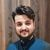# QlikView App Dev

Discussion Board for collaboration related to QlikView App Development.

cancel
Showing results for
Did you mean:Creator II

## Negation in set analysis

Hello All,

I am working on one solution where I have to implement one straight table With Date & UserID as first two columns.

Now, I have one button from which one value(Value1) is passed on selection.

So for my UserID i have to use Calculated Dimension as:

=If(Value1='Y', Only({<UserID -= {"Field1=Y OR Field2 =Y "} UserID>}), UserID)

I am sure that above syntax in incorrect but the logic should be that way. Can you please help.

Labels (1)
• ### Visualization

4 RepliesMVP

Could you prepare a sample app and share here explaining the expected output in that context?Creator II
Author

I cant post an app currently.  The Output should be Total population but removing UserIDs where Field1=Y & Field2=Y when Value1=Y otherwise it should contain whole population.MVP

As per your expression, you could try something like:

=If(Value1='Y', Aggr(Only({<UserID = e({<Field1={Y}>+<Field2 ={Y}> })>}, UserID),UserID), UserID)Specialist III

or add a similar calculation in EXPRESSION instead of a calculated dimension and hide the column:

=if(Value='Y',
sum({<UserID=e( {<Field1={'Y'}> + <Field2={'Y'}>}UserID)>}1),
sum(1))

and add UserID as a dimensionCommunity Browser